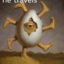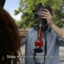MathematicsOpenStudy (anonymous):

3x-2y=5 -6x+4y=7 Solve the linear equation algebraically!OpenStudy (anonymous):

|dw:1314379656411:dw|OpenStudy (anonymous):OpenStudy (anonymous):

try using substitution or eliminationOpenStudy (anonymous):

i know that. i dont understand HOW to use it though.OpenStudy (anonymous):

okay take one of the equations, anyone and make it equal x or y ur choice. for ex: 3x-2y=5 +2y =2y 3x=5+2y /3 /3 x=5/3+2/3y Now take the other equation and subsitute the x in that equation for 5/3+2/3y and solve.OpenStudy (anonymous):

3x-2y=5..................(1) -6x+4y=7............(2) y=(-5+3x)/2 [ from equation 1] sub y in (2) -6x+4[(-5+3x)/2=7 0=17this means x is contained in empty set hence the system of linear equations is inconsistence(no solution)

Latest QuestionsShelbiLW: A 3.0 m uniform beam of mass 15 kg is pivoted 1.0 m from the end as shown below. A 35 kg child sits 0.
5 hours ago 0 Replies 0 Medalsryleighmullins22: Answer the question below using the following amortization table. Month Principal Paid Interest Paid Balance 21 \$654.
5 hours ago 0 Replies 0 MedalsTonycoolkid21: help
5 hours ago 8 Replies 0 MedalsTonycoolkid21: help
6 hours ago 15 Replies 3 MedalsRx1nyDxyz: Help If the supply of a product increases, then the price decreases*** the price
7 hours ago 21 Replies 1 Medalshototodorokii: (not from a test/quiz)Nomenclature is governed by certain universal rules. Which
7 hours ago 0 Replies 0 Medalscrispyrat: A guide to sqrt and cbrt
6 hours ago 21 Replies 3 MedalsxXQuintonXx: help please(ss below)
8 hours ago 5 Replies 1 Medalajfknkjfa: Prove that the two circles shown below are similar. (10 points) Circle C is shown with a center at negative 3, 1 and a radius of 4.
8 hours ago 3 Replies 1 Medalrxcklesskaisher: idk wdh dis is
8 hours ago 2 Replies 0 Medals